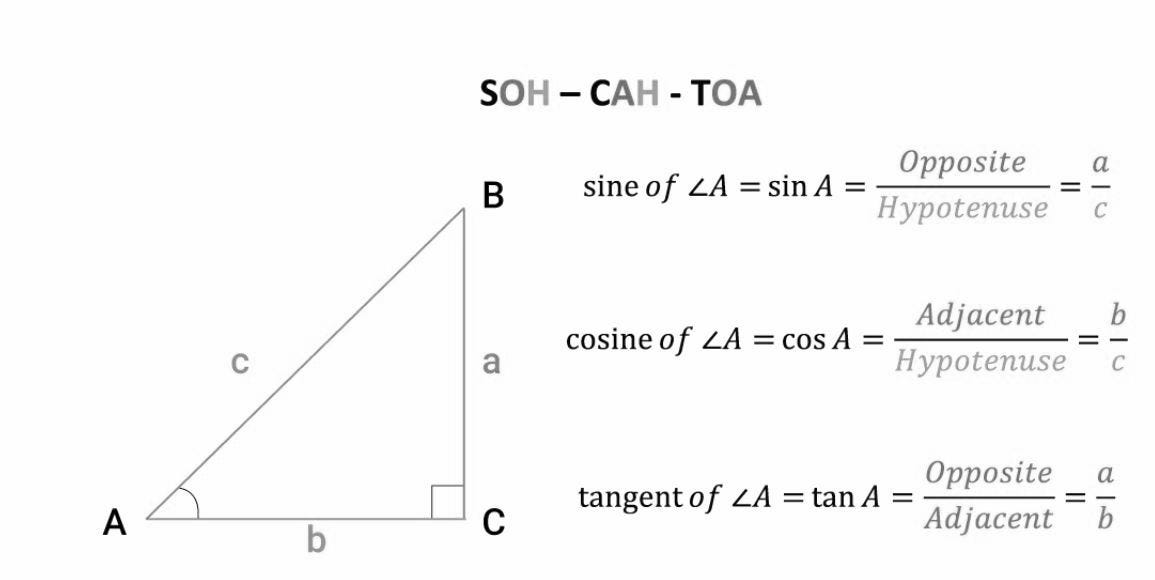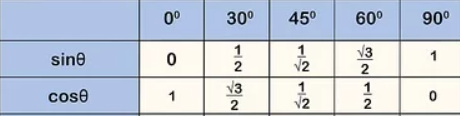# A deep dive into Principal Component Analysis (PCA)In this post, we are going to have an in-depth look at another popular unsupervised learning algorithm called Principal Component Analysis (PCA).

There are three steps to understand Principal Component Analysis: Understanding the Mathematics required for PCA, the Statistics concepts we would need and finally connect all the dots while solving the final Optimization Problem for PCA.

## Mathematics for PCA:

Pardon me, because I am going to take you back to high school Mathematics ! So, how much Math do we actually need to understand Principal Component Analysis ?

The answer is : Concepts of Linear Algebra and a few trigonometry rules should be good enough to dive-in into PCA concepts. Let’s start with few basic equations of trigonometry.

Trigonometry Rules you should keep handy:

We will be using cosine of angles quite frequently, hence we need to be very quick with the following rule for a right angled triangle.Second fundamental rule of trigonometry that we need to know to understand the upcoming steps is: Cos(A-B)=CosACosB + SinASinB.

Finally here is a quick table of all the sine & cosine angles.(I would encourage the readers to revisit the basic formulations of trigonometry and their corresponding proofs)Linear Algebra : Vectors

Now since we know the basics of trigonometry, let us walkthrough the linear algebra pre-requisites. We will base the entire theory of linear algebra onto vectors, so it becomes very crucial to be able to visualize them. Any vector in nD space is represented by the “n” coordinates. Each vector has a direction in the space and a related magnitude.

## Difference between Machine Learning, Data Science, AI, Deep Learning, and Statistics

In this article, I clarify the various roles of the data scientist, and how data science compares and overlaps with related fields such as machine learning, deep learning, AI, statistics, IoT, operations research, and applied mathematics.

## Statistics for Data Science

Statistics for Data Science and Machine Learning Engineer. I’ll try to teach you just enough to be dangerous, and pique your interest just enough that you’ll go off and learn more.

## Most popular Data Science and Machine Learning courses — July 2020

Most popular Data Science and Machine Learning courses — August 2020. This list was last updated in August 2020 — and will be updated regularly so as to keep it relevant

## 15 Machine Learning and Data Science Project Ideas with Datasets

Learning is a new fun in the field of Machine Learning and Data Science. In this article, we’ll be discussing 15 machine learning and data science projects.

## Best Free Datasets for Data Science and Machine Learning Projects

This post will help you in finding different websites where you can easily get free Datasets to practice and develop projects in Data Science and Machine Learning.